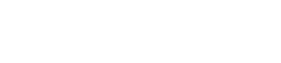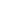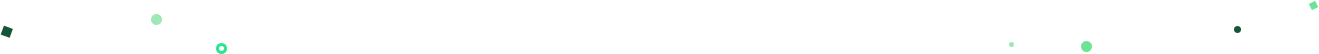NOW LIVEEmpower your end users with Explorations in Mode.Try it now## Mode Studio

The Collaborative Data Science Platform# SQL Comparison Operators

Starting here? This lesson is part of a full-length tutorial in using SQL for Data Analysis. Check out the beginning.

In this lesson we'll cover:

## Comparison operators on numerical data

The most basic way to filter data is using comparison operators. The easiest way to understand them is to start by looking at a list of them:

 Equal to `=` Not equal to `<>` or `!=` Greater than `>` Less than `<` Greater than or equal to `>=` Less than or equal to `<=`

These comparison operators make the most sense when applied to numerical columns. For example, let's use `>` to return only the rows where the West Region produced more than 30,000 housing units (remember, the units in this data table are already in thousands):

``````SELECT *
FROM tutorial.us_housing_units
WHERE west > 30
``````

Try running that query with each of the operators in place of `>`. Try some values other than `30` to get a sense of how SQL operators work. When you're ready, try out the practice problems.

## Practice Problem

Did the West Region ever produce more than 50,000 housing units in one month?

Try it out See the answer

## Practice Problem

Did the South Region ever produce 20,000 or fewer housing units in one month?

Try it out See the answer

## Comparison operators on non-numerical data

All of the above operators work on non-numerical data as well. `=` and `!=` make perfect sense—they allow you to select rows that match or don't match any value, respectively. For example, run the following query and you'll notice that none of the January rows show up:

``````SELECT *
FROM tutorial.us_housing_units
WHERE month_name != 'January'
``````

There are some important rules when using these operators, though. If you're using an operator with values that are non-numeric, you need to put the value in single quotes: `'value'`.

Note: SQL uses single quotes to reference column values.

You can use `>`, `<`, and the rest of the comparison operators on non-numeric columns as well—they filter based on alphabetical order. Try it out a couple times with different operators:

``````SELECT *
FROM tutorial.us_housing_units
WHERE month_name > 'January'
``````

If you're using `>`, `<`, `>=`, or `<=`, you don't necessarily need to be too specific about how you filter. Try this:

``````SELECT *
FROM tutorial.us_housing_units
WHERE month_name > 'J'
``````

The way SQL treats alphabetical ordering is a little bit tricky. You may have noticed in the above query that selecting `month_name > 'J'` will yield only rows in which `month_name` starts with "j" or later in the alphabet. "Wait a minute," you might say. "January is included in the results—shouldn't I have to use `month_name >= 'J'` to make that happen?" SQL considers 'Ja' to be greater than 'J' because it has an extra letter. It's worth noting that most dictionaries would list 'Ja' after 'J' as well.

## Practice Problem

Write a query that only shows rows for which the month name is February.

Try it out See the answer

## Practice Problem

Write a query that only shows rows for which the `month_name` starts with the letter "N" or an earlier letter in the alphabet.

Try it out See the answer

## Arithmetic in SQL

You can perform arithmetic in SQL using the same operators you would in Excel: `+`, `-`, `*`, `/`. However, in SQL you can only perform arithmetic across columns on values in a given row. To clarify, you can only add values in multiple columns from the same row together using `+`—if you want to add values across multiple rows, you'll need to use aggregate functions, which are covered in the Intermediate SQL section of this tutorial.

The example below illustrates the use of `+`:

``````SELECT year,
month,
west,
south,
west + south AS south_plus_west
FROM tutorial.us_housing_units
``````

The above example produces a column showing the sum of whatever is in the `south` and `west` columns for each row. You can chain arithmetic functions, including both column names and actual numbers:

``````SELECT year,
month,
west,
south,
west + south - 4 * year AS nonsense_column
FROM tutorial.us_housing_units
``````

The columns that contain the arithmetic functions are called "derived columns" because they are generated by modifying the information that exists in the underlying data.

## Practice Problem

Write a query that calculates the sum of all four regions in a separate column.

Try it out See the answer

As in Excel, you can use parentheses to manage the order of operations. For example, if you wanted to average the `west` and `south` columns, you could write something like this:

``````SELECT year,
month,
west,
south,
(west + south)/2 AS south_west_avg
FROM tutorial.us_housing_units
``````

It occasionally makes sense to use parentheses even when it's not absolutely necessary just to make your query easier to read.

## Practice Problem

Write a query that returns all rows for which more units were produced in the West region than in the Midwest and Northeast combined.

Try it out See the answer

## Practice Problem

Write a query that calculates the percentage of all houses completed in the United States represented by each region. Only return results from the year 2000 and later.

Hint: There should be four columns of percentages.

Try it out See the answer

Next Lesson

SQL Logical Operators# Get our weekly data newsletter

Work-related distractions for every data enthusiast.